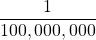Chapter 2. Measurements

# End-of-Chapter Material

1. Evaluate 0.00000000552 × 0.0000000006188 and express the answer in scientific notation. You may have to rewrite the original numbers in scientific notation first.
2. Evaluate 333,999,500,000 ÷ 0.00000000003396 and express the answer in scientific notation. You may need to rewrite the original numbers in scientific notation first.
3. Express the number 6.022 × 1023 in standard notation.
4. Express the number 6.626 × 10−34 in standard notation.
5. When powers of 10 are multiplied together, the powers are added together. For example,
102 × 103 = 102+3 = 105. With this in mind, can you evaluate (4.506 × 104) × (1.003 × 102) without entering scientific notation into your calculator?
6. When powers of 10 are divided into each other, the bottom exponent is subtracted from the top exponent. For example, 105 ÷ 103 = 105−3 = 102. With this in mind, can you evaluate
(8.552 × 106) ÷ (3.129 × 103) without entering scientific notation into your calculator?
7. Consider the quantity two dozen eggs. Is the number in this quantity “two” or “two dozen”? Justify your choice.
8. Consider the quantity two dozen eggs. Is the unit in this quantity “eggs” or “dozen eggs”? Justify your choice.
9. Fill in the blank: 1 km = ______________ μm.
10. Fill in the blank: 1 Ms = ______________ ns.
11. Fill in the blank: 1 cL = ______________ ML.
12. Fill in the blank: 1 mg = ______________ kg.
13. Express 67.3 km/h in metres/second.
14. Express 0.00444 m/s in kilometres/hour.
15. Using the idea that 1.602 km = 1.000 mi, convert a speed of 60.0 mi/h into kilometres/hour.
16. Using the idea that 1.602 km = 1.000 mi, convert a speed of 60.0 km/h into miles/hour.
17. Convert 52.09 km/h into metres/second.
18. Convert 2.155 m/s into kilometres/hour.
19. Use the formulas for converting degrees Fahrenheit into degrees Celsius to determine the relative size of the Fahrenheit degree over the Celsius degree.
20. Use the formulas for converting degrees Celsius into kelvins to determine the relative size of the Celsius degree over kelvins.
21. What is the mass of 12.67 L of mercury?
22. What is the mass of 0.663 m3 of air?
23. What is the volume of 2.884 kg of gold?
24. What is the volume of 40.99 kg of cork? Assume a density of 0.22 g/cm3.

1. 3.42 × 10−18
1. 602,200,000,000,000,000,000,000
1. 4.520 × 106
1. The quantity is two; dozen is the unit.
1. 1,000,000,000
1.1. 18.7 m/s
1. 96.1 km/h
1. 14.47 m/s
1. One Fahrenheit degree is nine-fifths the size of a Celsius degree.
1. 1.72 × 105 g
1. 149 mL